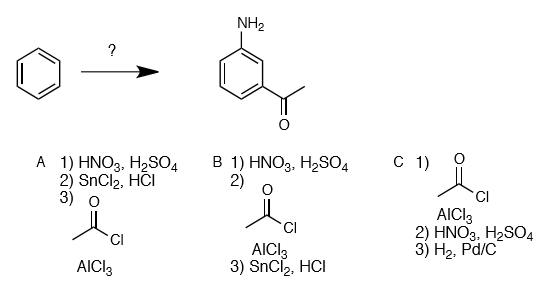# Problem: Which sequence of reactions would give (some of) the correct product?  1) A and B 2) A and C 3) B and C 4) B only 5) A, B, and C

###### FREE Expert Solution
98% (373 ratings)
###### Problem Details

Which sequence of reactions would give (some of) the correct product?

1) A and B

2) A and C

3) B and C

4) B only

5) A, B, and CWhat scientific concept do you need to know in order to solve this problem?

Our tutors have indicated that to solve this problem you will need to apply the EAS: Retrosynthesis concept. You can view video lessons to learn EAS: Retrosynthesis. Or if you need more EAS: Retrosynthesis practice, you can also practice EAS: Retrosynthesis practice problems.

What is the difficulty of this problem?

Our tutors rated the difficulty ofWhich sequence of reactions would give (some of) the correct...as high difficulty.

How long does this problem take to solve?

Our expert Organic tutor, Jonathan took 3 minutes and 24 seconds to solve this problem. You can follow their steps in the video explanation above.

What professor is this problem relevant for?

Based on our data, we think this problem is relevant for Professor Lever's class at MIZZOU.